#DAY 15
3

## Excel VBA 的眉眉角角Day15:關於寫入純文字檔的這檔事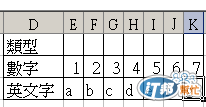``````Sub Day15_1()
Sheets("Day15").Select
Dim Rng As Object
Dim strData

'使用 Open 陳述式，配合Print寫入資料
'資料相連接的功能不是很完整，數字會補上左右邊空白

Open "D:\Day15_1.txt" For Output As #1

For Each Rng In Range("D2:K3")
If Rng.Column = 4 Then
Print #1, Rng, '兩欄位用空白隔開
ElseIf Rng.Column = 11 Then
Print #1, Rng   '自動換列
Else
Print #1, Rng;  '資料會相連接
End If
Next
Close #1
End Sub
``````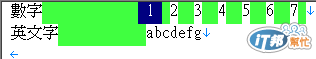``````Sub Day15_1_FIX()
Sheets("Day15").Select
Dim Rng As Object
Dim strData

'使用 Open 陳述式，配合Print寫入資料
'使用&連接自訂的「相隔符號」，取代內建的功能

Open "D:\Day15_1_FIX.txt" For Output As #1

For Each Rng In Range("D2:K3")
If Rng.Column = 4 Then
Print #1, Rng & vbTab;     '兩欄位用Tab隔開
ElseIf Rng.Column = 11 Then
Print #1, Rng & "" & vbCrLf;   '自動換列
Else
Print #1, Rng & "";   '資料會相連接
End If
Next
Close #1
End Sub
``````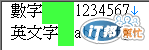``````Sub Day15_2()
Sheets("Day15").Select
Dim Rng As Object
Dim strData As String

For Each Rng In Range("D2:K3")
If Rng.Column = 4 Then
strData = strData & Rng & vbTab
ElseIf Rng.Column = 11 Then
strData = strData & Rng & vbCrLf
Else
strData = strData & Rng
End If
Next
Debug.Print strData

Open "D:\Day15_2.txt" For Output As #1
Print #1, strData
Close #1
End Sub
``````

``````Sub Day15_3_1()
Sheets("Day15").Select
Dim Rng As Object
Dim strData

Open "D:\Day15_3_1.txt" For Output As #1

For Each Rng In Range("D2:K3")
If Rng.Column = 4 Then
Write #1, Rng,  '塞入空白，沒效，會出現逗點
ElseIf Rng.Column = 11 Then
Write #1, Rng   '換行，可以
Else
Write #1, Rng;  '連接，沒效，會出現逗點分隔
End If
Next

Close #1

End Sub
``````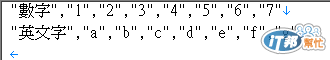``````Sub Day15_3_2 ()
Sheets("Day15").Select
Dim Rng As Object
Dim strData As String

For Each Rng In Range("D2:K3")
If Rng.Column = 4 Then
strData = strData & Rng & vbTab
ElseIf Rng.Column = 11 Then
strData = strData & Rng & vbCrLf
Else
strData = strData & Rng
End If
Next
Debug.Print strData

Open "D:\ Day15_3_2.txt" For Output As #1
Write #1, strData
Close #1
End Sub
``````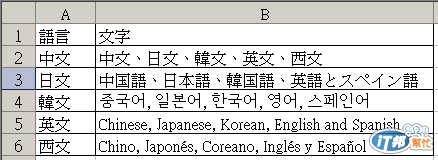``````Sub Day15_4_1()
'多國語言文字寫入ASCII格式的文字檔
Sheets("Day15").Select
Dim Rng As Object
Dim strData

For Each Rng In Range("A1:B6")
If Rng.Column = 1 Then
strData = strData & Rng & vbTab
ElseIf Rng.Column = 2 Then
strData = strData & Rng & vbCrLf
End If
Next
Debug.Print strData

Open "D:\Day15_4_1.txt" For Output As #1
Print #1, strData
Close #1
End Sub
``````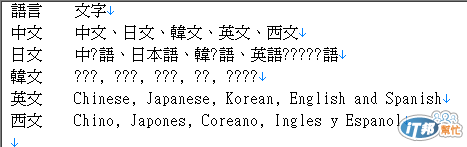``````Sub Day15_4_2()
'多國語言文字寫入UTF8格式的文字檔
Sheets("Day15").Select
Dim Rng As Object
Dim strData

For Each Rng In Range("A1:B6")
If Rng.Column = 1 Then
strData = strData & Rng & vbTab
ElseIf Rng.Column = 2 Then
strData = strData & Rng & vbCr
End If
Next
Debug.Print strData

Dim fsT As Object
fsT.Type = 2 '指定類型，儲存文字資料使用2
fsT.Charset = "UTF-8" '指定字元集為UTF8
fsT.Open '開啟與寫入二進位資料到物件
fsT.WriteText strData
fsT.SaveToFile "D:\Day15_4_2.txt", 2 '寫入二進位資料到磁碟
End Sub
``````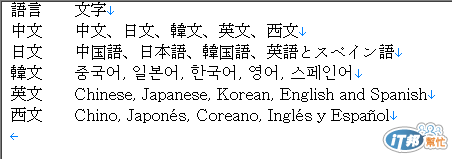Excel VBA 的眉眉角角30